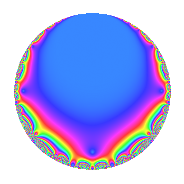Properties

 Label 57.2.a.aLevel 57 Weight 2 Character orbit 57.a Self dual Yes Analytic conductor 0.455 Analytic rank 1 Dimension 1 CM No Inner twists 1

Related objects

Newspace parameters

 Level: $$N$$ = $$57 = 3 \cdot 19$$ Weight: $$k$$ = $$2$$ Character orbit: $$[\chi]$$ = 57.a (trivial)

Newform invariants

 Self dual: Yes Analytic conductor: $$0.455147291521$$ Analytic rank: $$1$$ Dimension: $$1$$ Coefficient field: $$\mathbb{Q}$$ Coefficient ring: $$\mathbb{Z}$$ Coefficient ring index: $$1$$ Fricke sign: $$1$$ Sato-Tate group: $\mathrm{SU}(2)$

$q$-expansion

 $$f(q)$$ $$=$$ $$q$$ $$\mathstrut -\mathstrut 2q^{2}$$ $$\mathstrut -\mathstrut q^{3}$$ $$\mathstrut +\mathstrut 2q^{4}$$ $$\mathstrut -\mathstrut 3q^{5}$$ $$\mathstrut +\mathstrut 2q^{6}$$ $$\mathstrut -\mathstrut 5q^{7}$$ $$\mathstrut +\mathstrut q^{9}$$ $$\mathstrut +\mathstrut O(q^{10})$$ $$q$$ $$\mathstrut -\mathstrut 2q^{2}$$ $$\mathstrut -\mathstrut q^{3}$$ $$\mathstrut +\mathstrut 2q^{4}$$ $$\mathstrut -\mathstrut 3q^{5}$$ $$\mathstrut +\mathstrut 2q^{6}$$ $$\mathstrut -\mathstrut 5q^{7}$$ $$\mathstrut +\mathstrut q^{9}$$ $$\mathstrut +\mathstrut 6q^{10}$$ $$\mathstrut +\mathstrut q^{11}$$ $$\mathstrut -\mathstrut 2q^{12}$$ $$\mathstrut +\mathstrut 2q^{13}$$ $$\mathstrut +\mathstrut 10q^{14}$$ $$\mathstrut +\mathstrut 3q^{15}$$ $$\mathstrut -\mathstrut 4q^{16}$$ $$\mathstrut -\mathstrut q^{17}$$ $$\mathstrut -\mathstrut 2q^{18}$$ $$\mathstrut -\mathstrut q^{19}$$ $$\mathstrut -\mathstrut 6q^{20}$$ $$\mathstrut +\mathstrut 5q^{21}$$ $$\mathstrut -\mathstrut 2q^{22}$$ $$\mathstrut -\mathstrut 4q^{23}$$ $$\mathstrut +\mathstrut 4q^{25}$$ $$\mathstrut -\mathstrut 4q^{26}$$ $$\mathstrut -\mathstrut q^{27}$$ $$\mathstrut -\mathstrut 10q^{28}$$ $$\mathstrut -\mathstrut 2q^{29}$$ $$\mathstrut -\mathstrut 6q^{30}$$ $$\mathstrut -\mathstrut 6q^{31}$$ $$\mathstrut +\mathstrut 8q^{32}$$ $$\mathstrut -\mathstrut q^{33}$$ $$\mathstrut +\mathstrut 2q^{34}$$ $$\mathstrut +\mathstrut 15q^{35}$$ $$\mathstrut +\mathstrut 2q^{36}$$ $$\mathstrut +\mathstrut 2q^{38}$$ $$\mathstrut -\mathstrut 2q^{39}$$ $$\mathstrut -\mathstrut 10q^{42}$$ $$\mathstrut -\mathstrut q^{43}$$ $$\mathstrut +\mathstrut 2q^{44}$$ $$\mathstrut -\mathstrut 3q^{45}$$ $$\mathstrut +\mathstrut 8q^{46}$$ $$\mathstrut -\mathstrut 9q^{47}$$ $$\mathstrut +\mathstrut 4q^{48}$$ $$\mathstrut +\mathstrut 18q^{49}$$ $$\mathstrut -\mathstrut 8q^{50}$$ $$\mathstrut +\mathstrut q^{51}$$ $$\mathstrut +\mathstrut 4q^{52}$$ $$\mathstrut +\mathstrut 10q^{53}$$ $$\mathstrut +\mathstrut 2q^{54}$$ $$\mathstrut -\mathstrut 3q^{55}$$ $$\mathstrut +\mathstrut q^{57}$$ $$\mathstrut +\mathstrut 4q^{58}$$ $$\mathstrut -\mathstrut 8q^{59}$$ $$\mathstrut +\mathstrut 6q^{60}$$ $$\mathstrut -\mathstrut q^{61}$$ $$\mathstrut +\mathstrut 12q^{62}$$ $$\mathstrut -\mathstrut 5q^{63}$$ $$\mathstrut -\mathstrut 8q^{64}$$ $$\mathstrut -\mathstrut 6q^{65}$$ $$\mathstrut +\mathstrut 2q^{66}$$ $$\mathstrut +\mathstrut 8q^{67}$$ $$\mathstrut -\mathstrut 2q^{68}$$ $$\mathstrut +\mathstrut 4q^{69}$$ $$\mathstrut -\mathstrut 30q^{70}$$ $$\mathstrut -\mathstrut 12q^{71}$$ $$\mathstrut -\mathstrut 11q^{73}$$ $$\mathstrut -\mathstrut 4q^{75}$$ $$\mathstrut -\mathstrut 2q^{76}$$ $$\mathstrut -\mathstrut 5q^{77}$$ $$\mathstrut +\mathstrut 4q^{78}$$ $$\mathstrut +\mathstrut 16q^{79}$$ $$\mathstrut +\mathstrut 12q^{80}$$ $$\mathstrut +\mathstrut q^{81}$$ $$\mathstrut +\mathstrut 12q^{83}$$ $$\mathstrut +\mathstrut 10q^{84}$$ $$\mathstrut +\mathstrut 3q^{85}$$ $$\mathstrut +\mathstrut 2q^{86}$$ $$\mathstrut +\mathstrut 2q^{87}$$ $$\mathstrut -\mathstrut 6q^{89}$$ $$\mathstrut +\mathstrut 6q^{90}$$ $$\mathstrut -\mathstrut 10q^{91}$$ $$\mathstrut -\mathstrut 8q^{92}$$ $$\mathstrut +\mathstrut 6q^{93}$$ $$\mathstrut +\mathstrut 18q^{94}$$ $$\mathstrut +\mathstrut 3q^{95}$$ $$\mathstrut -\mathstrut 8q^{96}$$ $$\mathstrut -\mathstrut 10q^{97}$$ $$\mathstrut -\mathstrut 36q^{98}$$ $$\mathstrut +\mathstrut q^{99}$$ $$\mathstrut +\mathstrut O(q^{100})$$

Embeddings

For each embedding $$\iota_m$$ of the coefficient field, the values $$\iota_m(a_n)$$ are shown below.

For more information on an embedded modular form you can click on its label.

Label $$\iota_m(\nu)$$ $$a_{2}$$ $$a_{3}$$ $$a_{4}$$ $$a_{5}$$ $$a_{6}$$ $$a_{7}$$ $$a_{8}$$ $$a_{9}$$ $$a_{10}$$
1.1
 0
−2.00000 −1.00000 2.00000 −3.00000 2.00000 −5.00000 0 1.00000 6.00000
 $$n$$: e.g. 2-40 or 990-1000 Significant digits: Format: Complex embeddings Normalized embeddings Satake parameters Satake angles

Inner twists

This newform does not admit any (nontrivial) inner twists.

Atkin-Lehner signs

$$p$$ Sign
$$3$$ $$1$$
$$19$$ $$1$$

Hecke kernels

This newform can be constructed as the intersection of the kernels of the following linear operators acting on $$S_{2}^{\mathrm{new}}(\Gamma_0(57))$$:

 $$T_{2}$$ $$\mathstrut +\mathstrut 2$$ $$T_{5}$$ $$\mathstrut +\mathstrut 3$$